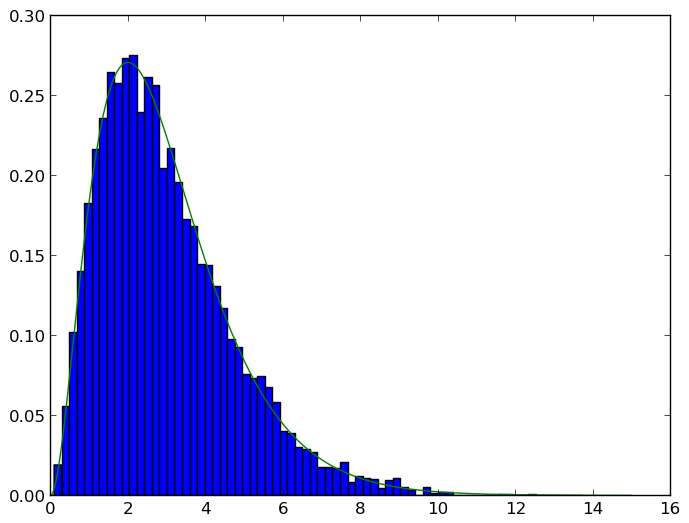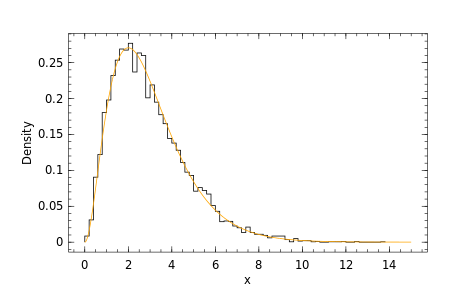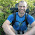## Tuesday, July 22, 2014

### Plotting probability distributions in Julia

I often find the need to plot out probability distributions of parameters, for example posterior distributions. There are lots of ways to do this in Julia, and a clear 'winner' for plotting has yet to be established (although there's always room for implementations). Here, I outline some of the ways to generate probability histograms.

To generate the data, I first need to load Distributions and DataFrames.

In :
using Distributions
using DataFrames;


There doesn't appear to be a function in the standard library to normalise histograms, so here is a function to calculate the total area under the curve for a histogram, using the tuple returned by the hist function.

In :
function auc(h::(Range{Float64},Array{Int64,1}))
freq=convert(Array{Float64,1},h)
e=convert(Array{Float64,1},h)
numbins=length(e)
deltax=e[2:numbins]-e[1:(numbins-1)]
sum(deltax.*freq)
end;


I now generate some random data from a $Gamma(3,1)$ distribution.

In :
srand(1)
x=rand(Distributions.Gamma(3,1),10000)
xhist=hist(x,100)
nbins=length(xhist)
xauc=auc(xhist)
xvec=vec([z::Float64 for z in xhist])
xdf=DataFrame(xmin=vec(xvec[1:(nbins-1)]),xmax=vec(xvec[2:nbins]),count=xhist)
xdf[:dens]=xdf[:count]/xauc;


Here's what the data look like.

In :
xdf

Out:
xminxmaxcountdens
10.00.2170.0085
20.20.4620.031
30.40.61810.0905
40.60.82440.122
50.81.03610.1805
61.01.23960.198
71.21.44640.232
81.41.65070.2535
91.61.85380.269
101.82.05350.2675
112.02.25540.277
122.22.44740.237
132.42.65270.2635
142.62.85200.26
152.83.04020.201
163.03.24380.219
173.23.43900.195
183.43.63550.1775
193.63.83300.165
203.84.02890.1445
214.04.22760.138
224.24.42560.128
234.44.62220.111
244.64.81950.0975
254.85.01860.093
265.05.21420.071
275.25.41520.076
285.45.61440.072
295.65.81340.067
305.86.01020.051

I generate a dataframe containing points from the PDF, which I will use to compare the random values.

In :
xp=DataFrame(x=linspace(0,15,1501),dens=pdf(Distributions.Gamma(3,1),linspace(0,15,1501)))
xp

Out:
xdens
10.00.0
20.014.950249168745848e-5
30.020.00019603973466135092
40.030.0004367004900968286
50.040.0007686315513218585
60.050.0011890367806258926
70.060.0016951761604516473
80.070.0022843648587695725
90.080.0029539723084372345
100.090.0037014213003484743
110.10.004524187090179799
120.110.005419796518543995
130.120.006385827144363534
140.130.007419906391278744
150.140.008519710706908304
160.150.009682964734781897
170.160.010907440498767502
180.170.012190956599817747
190.180.013531377424862616
200.190.014926612367677677
210.20.016374615061559628
220.210.017873382623642627
230.220.019420954910691974
240.229999999999999980.021015413786213192
250.240.022654882398716738
260.250.024337524470981413
270.260.02606154360016055
280.269999999999999960.02782518256857831
290.280.02962672266506445
300.290000000000000040.031464483016678674

## PyPlot

As I use IJulia a lot, the most straightforward place to start is to use PyPlot, which is just an interface to Python's matplotlib. PyPlot has an option to normalise the histogram, so we pass the raw data, and PyPlot generates the histogram for us.

In :
using PyPlot
PyPlot.plt.hist(x,nbins,normed="True")
PyPlot.plt.plot(xp[:x],xp[:dens])

INFO: Loading help data...Out:
1-element Array{Any,1}:
PyObject <matplotlib.lines.Line2D object at 0xf43ee10>


Gadfly is one of the more polished plotting packages out there, and takes many of its cues from Hadley Wickham's excellent ggplot2 library. However, it seems that there isn't an option to normalise a histogram, so I use a barplot of the pre-computed densities instead. To get different colours for the histogram and the density plot, I use Themes.

In :
using Gadfly
layer(x=xp[:x],y=xp[:dens],Geom.line,Theme(default_color=color("orange"))))

Out:

## Winston

Winston is another nice plotting library, which is very easy to use. It can take the output of the hist command, as well as easily add a curve on top.

In :
using Winston
p = Winston.FramedPlot(title="", xlabel="x", ylabel="Density")
xhist2=(xhist,xhist./xauc)

Out:## Plotly

Plotly is an online service that generates some nice plots; one will need an account and an API key to be able to use it, however (fill in yours below). To install, you need to run Pkg.clone("https://github.com/plotly/Plotly.jl"). I also had to edit the URL, as I run my IJulia notebook over HTTPS, and Chrome doesn't like insecure content loaded from a secure site.

In :
using Plotly
api_key=""
data0 = ["x" => x, "type" => "histogramx", "histnorm" => "probability density"]
data1 = ["x" => xp["x"],"y" => xp["dens"], "type" => "scatter"]
layout = {
"title"=> "PDF",
"autosize"=> "false",
"width"=> 700,
"height"=> 700,
"titlefont"=>
{
"family"=> "Open Sans",
"size"=> 18,
"color"=> "rgb(84, 39, 143)"
},
"xaxis"=> {
"title"=> "x"
},
"yaxis"=> {"title"=> "Density"},
"showlegend" => false
};
response = Plotly.plot([data0, data1],["layout"=>layout])
url = response["url"]
url = replace(url,"http","https")
s = string("<iframe height='750' id='igraph' scrolling='no' seamless='seamless' src='",
url,
"/700/700' width='750'></iframe>")
display("text/html", s);

Warning: using Winston.text in module Main conflicts with an existing identifier.
WARNING: indexing DataFrames with strings is deprecated; use symbols instead
in getindex at /home/simon/.julia/v0.3/DataFrames/src/deprecated.jl:170
WARNING: indexing DataFrames with strings is deprecated; use symbols instead
in getindex at /home/simon/.julia/v0.3/DataFrames/src/deprecated.jl:170



## Conclusions

There are some other packages for plotting in Julia, like Gaston and Vega. Gaston is an interface to Gnuplot. Out of the box, it supports basic histograms, but if you want to do anything more complex, like add a line to a histogram, one has to drop down to the mid-level interface. In addition, Gaston doesn't currently support inline images in IJulia, and I've had problems with it recently. Vega has potential, but isn't as fully featured as some of the other plotting packages and hasn't been updated recently.

1.Nice post, Thanks!

2.The syntax for both histograms and tuples for arguments has changed as of Julia 0.5+, so the above code to generate the data doesn't work. Try the following:


using Distributions
using DataFrames

function auc(h::Tuple{Range{Float64},Array{Int64,1}})
freq=convert(Array{Float64,1},h)
e=convert(Array{Float64,1},h)
numbins=length(e)
deltax=e[2:numbins]-e[1:(numbins-1)]
sum(deltax.*freq)
end;

function auc(h::Histogram)
freq=convert(Array{Float64,1},h.weights)
e=convert(Array{Float64,1},h.edges)
numbins=length(e)
deltax=e[2:numbins]-e[1:(numbins-1)]
sum(deltax.*freq)
end;

srand(1)
x=rand(Distributions.Gamma(3,1),10000)

xhist=hist(x,100)
nbins=length(xhist)
xauc=auc(xhist)
xvec=vec([z::Float64 for z in xhist])
xdf=DataFrame(xmin=vec(xvec[1:(nbins-1)]),xmax=vec(xvec[2:nbins]),count=xhist)
xdf[:dens]=xdf[:count]/xauc

xhist2=fit(Histogram,x,nbins=100)
xauc2=auc(xhist2)
nbins2=length(xhist2.edges)
xvec2=vec([z::Float64 for z in xhist2.edges])
xdf2=DataFrame(xmin=vec(xvec2[1:(nbins-1)]),xmax=vec(xvec2[2:nbins]),count=xhist2.weights)
xdf2[:dens]=xdf2[:count]/xauc2


1.Great post! The hist function has been removed in Juia 0.6:

https://github.com/JuliaLang/julia/pull/16450

3.Nice post, thank you!